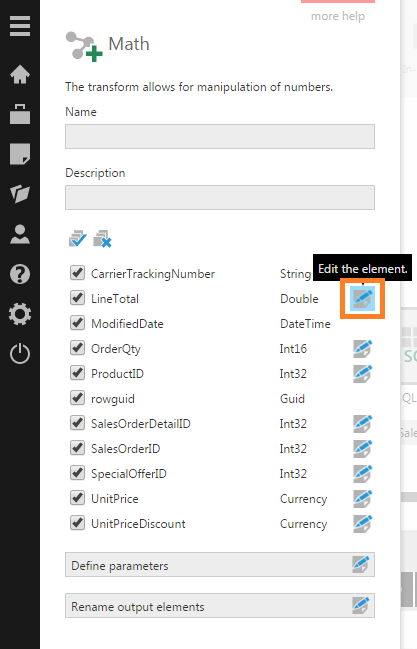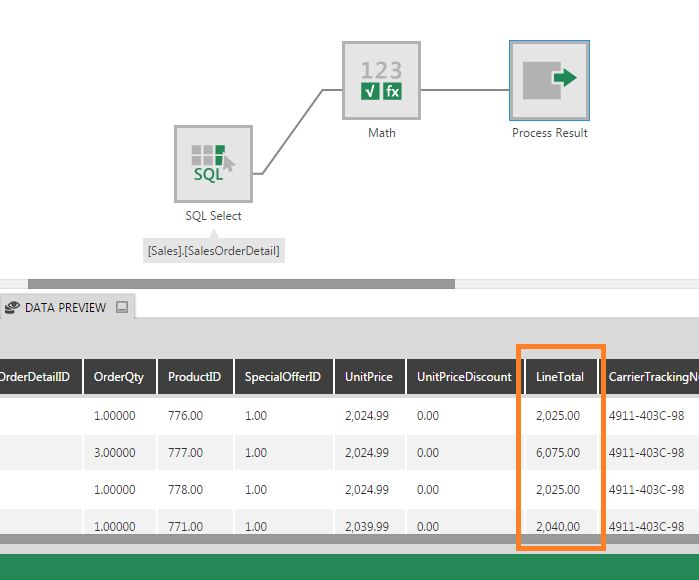# Math

Contents[Hide]

The Math transform lets you perform simple math functions such as Absolute, Round, and Square Root on numeric input columns. It is similar to the String transform except that it offers math functions instead of string functions.

The following functions are available:

• Round
• Absolute
• Ceiling
• Floor
• Truncate
• Square Root
• Multiply by 100

Tip
You can also apply formula functions by using a Calculated Element transform.

## 1. Input

The Math transform requires one input transform that has one or more numeric columns.

To add this transform to an existing data cube process, first select the connection link between two connected transforms.

Go to the toolbar, click Insert Common, and then select Math.

The Math transform is inserted between the two transforms.

## 3. Configure the transform

Double-click the Math transform or select the Configure option from its right-click menu.

In the configuration dialog for the transform, decide which input column you want to apply a math function on, and then click the column's Edit icon. Also uncheck any columns that should be excluded from the output.Edit a column if you want to apply a Math function

In the Transform Element dialog, use the dropdown to select the Math function you want (e.g., Round). Then click the submit button at the bottom of the dialog.

You can apply math functions to more than one numeric column with a single transform.

## 4. Output

The output of the Math transform consists of the columns that were checked, where the values in each numeric column with a math function selected are changed.

Click the Process Result transform and open the Data Preview to see the output of the transform.Output of Math transform shows LineTotal values have all been rounded